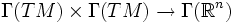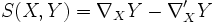# Second fundamental form

## Definition

Let$M$ be a manifold embedded in$\R^n$. the second fundamental form on$M$ is the map$\Gamma(TM) \times \Gamma(TM) \to \Gamma(\R^n)$ defined as follows:$S(X,Y) = \nabla_XY - \nabla'_XY$

where$\nabla$ si the Levi-Civita connection of$\R^n$ and$\nabla'$ is the Levi-Civita connection on$M$.